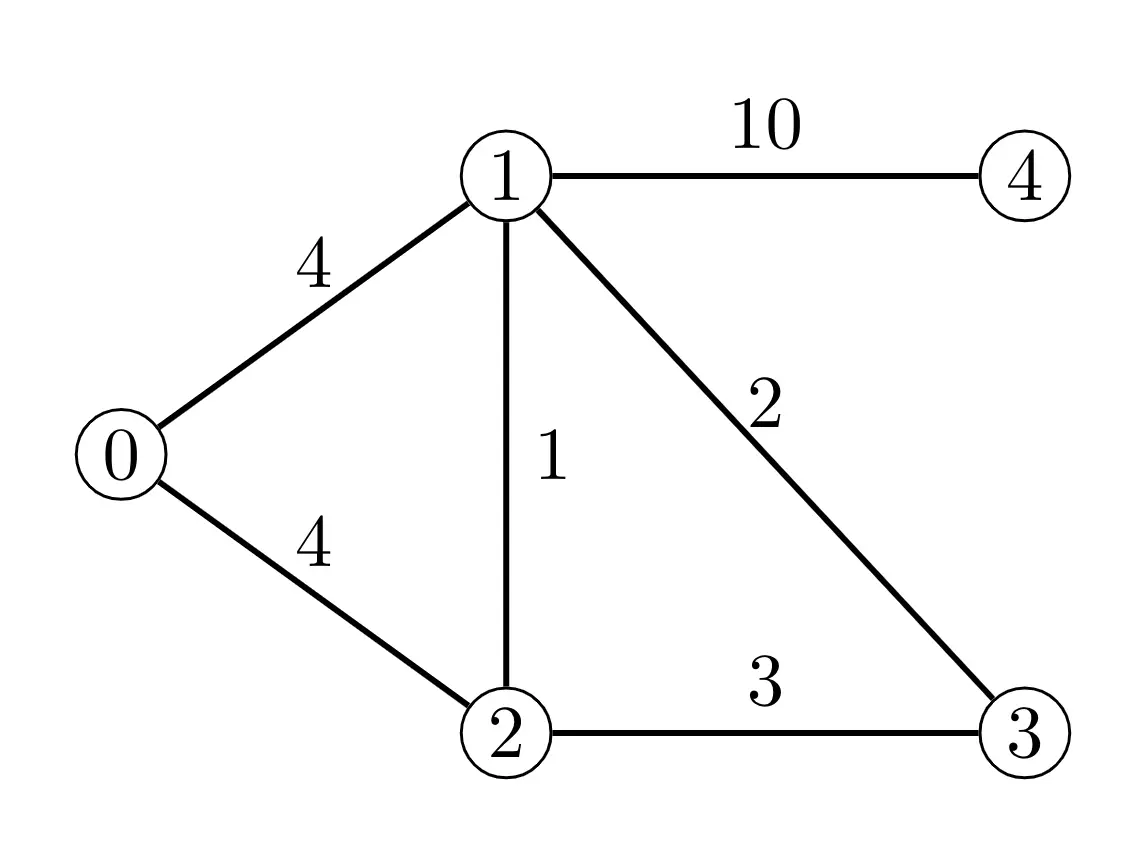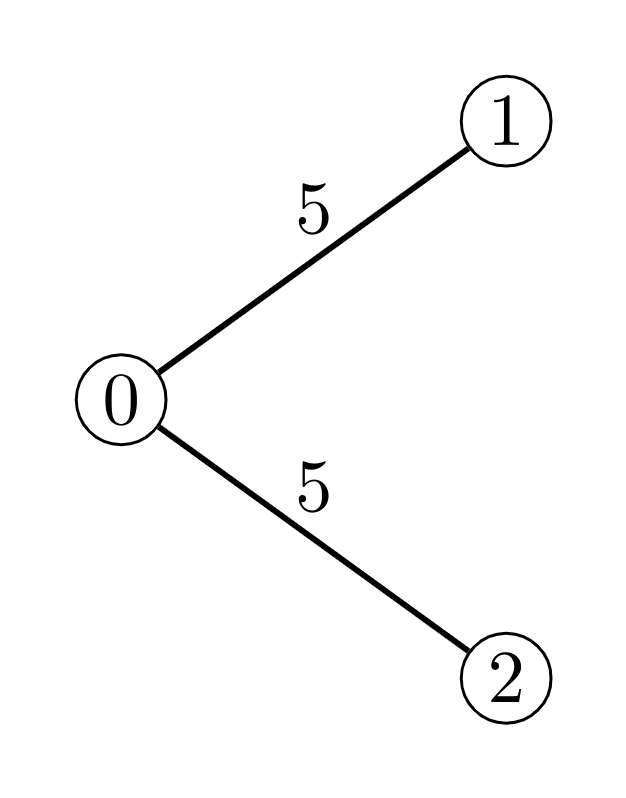# #556. 【APIO2020】交换城市

### 实现细节

void init(int N, int M, std::vector<int> U, std::vector<int> V, std::vector<int> W)
• $N$：一个整数表示城市数。
• $M$：一个整数表示道路数。
• $U$：一个长为 $M$ 的整数序列表示道路的第一个端点城市。
• $V$：一个长为 $M$ 的整数序列表示道路的第二个端点城市。
• $W$：一个长为 $M$ 的整数序列表示道路的汽油消耗。

int getMinimumFuelCapacity(int X, int Y)
• $X$：一个整数表示第一个城市。
• $Y$：一个整数表示第二个城市。
• 该函数必须返回一个整数，表示根据题目描述中的规则，两辆分别从第 $X$ 个城市与第 $Y$ 个城市出发要到达彼此城市的车，它们的单位汽油容量最大值的最小值。若无法满足题目规则则返回 $−1$。

### 样例评测库

N M
U V W
U V W
.
.
.
U[M-1] V[M-1] W[M-1]
Q
X Y
X Y
.
.
.
X[Q-1] Y[Q-1]

### 样例一

#### input

5 6
0 1 4
0 2 4
1 2 1
1 3 2
1 4 10
2 3 3
3
1 2
2 4
0 1



#### output

3
10
4



#### explanation### 样例二### 数据范围

• $2 \leq N \leq 10^5$
• $N − 1 \leq M \leq 2 \times 10^5$
• $0 \leq U[i] < V [i] < N$
• 任意两个城市间至多存在一条道路直接相连。
• 任意两个城市经过道路可以互相到达。
• $1 \leq W[i] \leq 10^9$
• $1 \leq Q \leq 2 \times 10^5$
• $0 \leq X[j] < Y [j] < N$

$1$ 每个城市至多是两条道路的一个端点。 $6$ 1, 2, 3, 4, 9
$2$ $M = N − 1, U[i] = 0$ $7$ 1, 5
$3$ $Q\le 5, N\le 1000, M\le 2000$ $17$ 1, 2, 6, 10
$4$ $Q\le 5$ $20$ 1, 2, 3, 6, 7, 10, 11
$5$ $M=N-1$ $23$ 1, 2, 3, 4, 5, 6, 7, 8
$6$  $27$ 1, 2, 3, 4, 5, 6, 7, 8, 9, 10, 11, 12